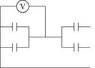# Very basic current direction question

• Jacob87411

#### Jacob87411

I attached a picture..Im just curious, if your calculating the capacitance on each plate why is the answer .5 if the V=1000 and each capacitor plate is 500 microfarads..

If C=500 for each plate then first you have two sets of parallel plates so it simples to a series with two plates of 1000 microfarads..which simplifies to 1/1000+1/1000=2/1000, so the capacitance is 500. C=Q/V,

5x10^-6=Q/1000, shouldn't Q=5? Thanks

Sorry now I attached it

#### Attachments

•image1.JPG
2.3 KB · Views: 259
Your attachment appears to be missing a connection to the rightmost part of the two right caps.

But assuming that there is meant to be a connection there, then you have basically 4 caps in parallel, with the way the voltage source is shown connected.

Yes they are supposed to be connected...so once you combine the parallel sets on either side and reduce it to two capacitors are those capacitors in parallel or series

The current has a choice of where to go

So it only flows through one pair of the capacitors, not both?

It can flow to both. Its just the matter of finding one equivalent capacitance now. Since you reduced the four parallel caps to two equivalent caps (one on each side), the current can go to either cap. When a current has more than one path to go, those components are in...?